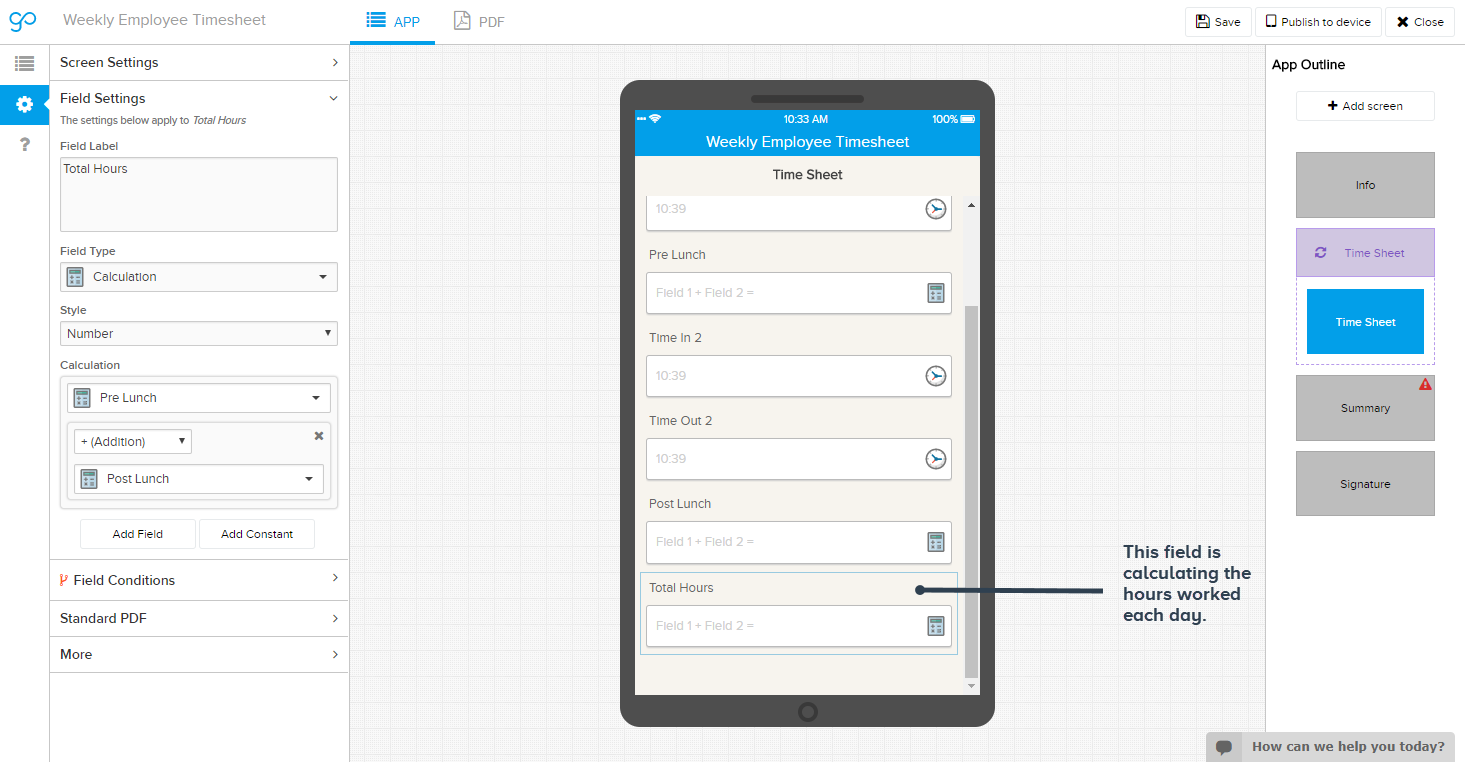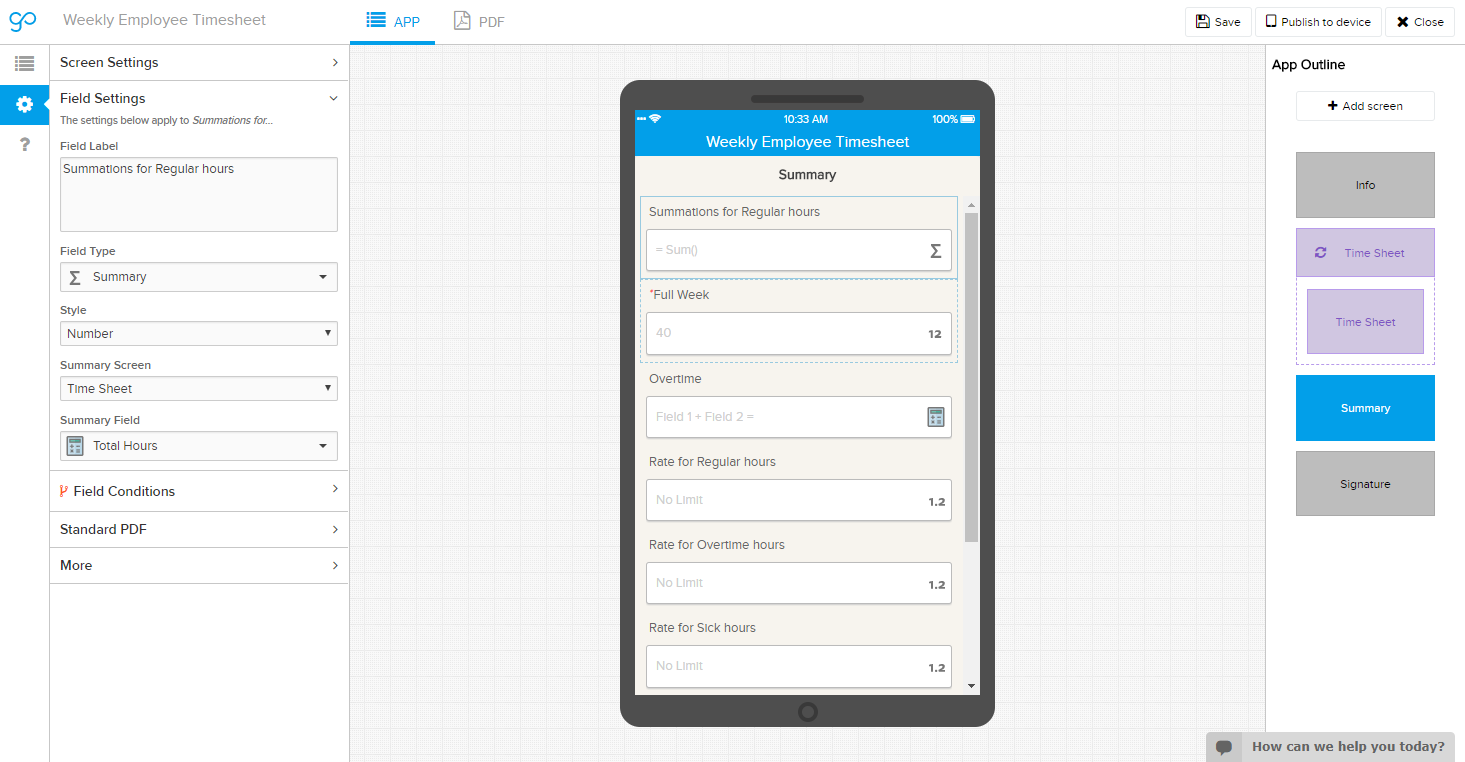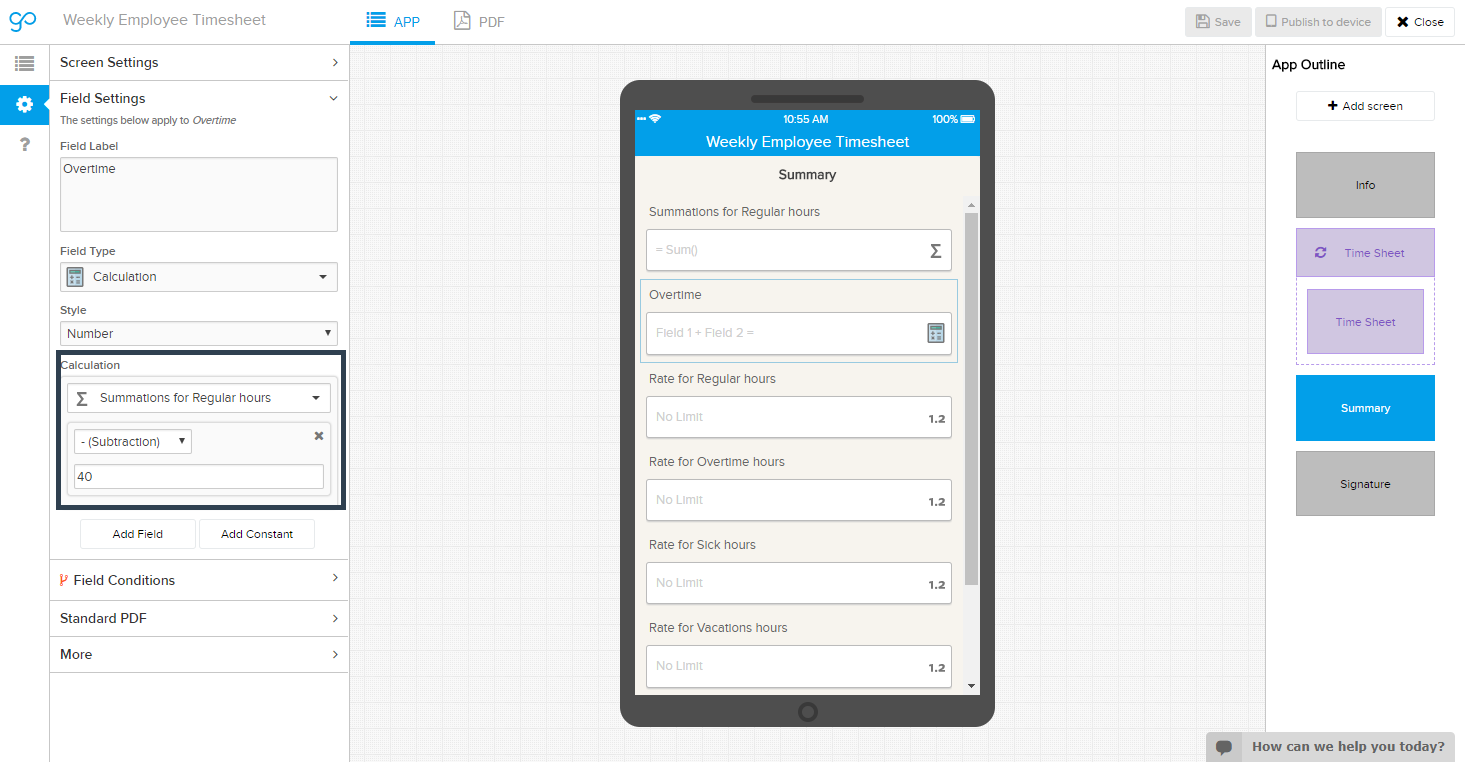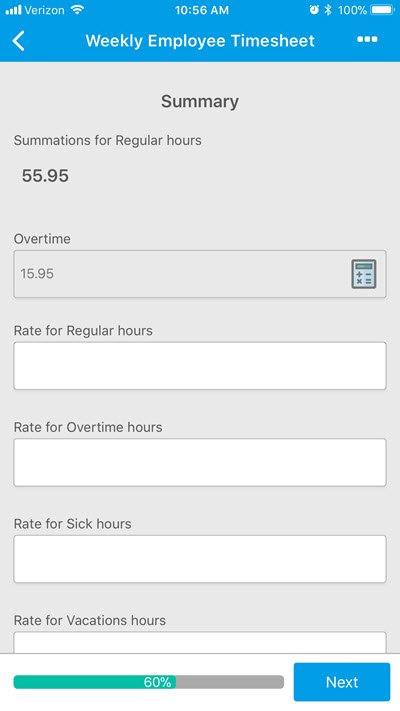•Sara Kaplow, Community Manager

Hi Lauren,

This is a great question. I think there are different ways to tackle it, but here's what I would do. This assumes that the calculation happens at the end of the week, and not on a daily basis.

I'd have a loop screen set up to get the info for each day. On each of those, you'd have a field for the total hours worked that day. Here's what that looks like (this is adding up the time from start until lunch, and then lunch through the end of the day):Then, outside of the loop, you'd want a Summary screen where you have a summary field getting you a total hours worked (called Summations for Regular Hours):Then you'll need a calculation field, set up to have the Summations for regular hours subtracting a constant, and that constant set to 40.That will give you the overtime hours worked that week.

Here's what that looks like on mobile:Does that satisfy what you're trying to do? I'd be happy to get some other brains on this if you have something more specific in mind.

Thanks!

•I will give this a try and let you know. Thank you!